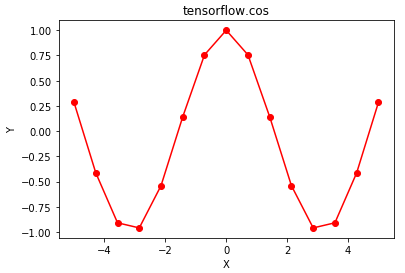GeeksforGeeks App
Open AppBrowser
Continue

# Python | Tensorflow cos() method

Tensorflow is an open-source machine learning library developed by Google. One of its applications is to develop deep neural networks.

The module tensorflow.math provides support for many basic mathematical operations. Function tf.cos() [alias tf.math.cos] provides support for the cosine function in Tensorflow. It expects the input in radian form and the output is in the range [-1, 1]. The input type is tensor and if the input contains more than one element, element-wise cosine is computed.

Syntax: tf.cos(x, name=None) or tf.math.cos(x, name=None)

Parameters
x: A tensor of any of the following types: float16, float32, float64, complex64, or complex128.
name (optional): The name for the operation.
Return type: A tensor with the same type as that of x.

Code #1:

## Python3

 `# Importing the Tensorflow library``import` `tensorflow as tf` `# A constant vector of size 6``a ``=` `tf.constant([``1.0``, ``-``0.5``, ``3.4``, ``-``2.1``, ``0.0``, ``-``6.5``], dtype ``=` `tf.float32)` `# Applying the cos function and``# storing the result in 'b'``b ``=` `tf.cos(a, name ``=``'cos'``)` `# Initiating a Tensorflow session``with tf.Session() as sess:``    ``print``(``'Input type:'``, a)``    ``print``(``'Input:'``, sess.run(a))``    ``print``(``'Return type:'``, b)``    ``print``(``'Output:'``, sess.run(b))`

Output:

```Input type: Tensor("Const_2:0", shape=(6, ), dtype=float32)
Input: [ 1.        -0.5        3.4000001 -2.0999999  0.        -6.5      ]
Return type: Tensor("cos:0", shape=(6, ), dtype=float32)
Output: [ 0.54030228  0.87758255 -0.96679819 -0.50484604  1.          0.97658765]```

Code #2: Visualization

## Python3

 `# Importing the Tensorflow library``import` `tensorflow as tf` `# Importing the NumPy library``import` `numpy as np` `# Importing the matplotlib.pyplot function``import` `matplotlib.pyplot as plt` `# A vector of size 15 with values from -5 to 5``a ``=` `np.linspace(``-``5``, ``5``, ``15``)` `# Applying the cos function and``# storing the result in 'b'``b ``=` `tf.cos(a, name ``=``'cos'``)` `# Initiating a Tensorflow session``with tf.Session() as sess:``    ``print``(``'Input:'``, a)``    ``print``(``'Output:'``, sess.run(b))``    ``plt.plot(a, sess.run(b), color ``=` `'red'``, marker ``=` `"o"``)``    ``plt.title(``"tensorflow.cos"``)``    ``plt.xlabel(``"X"``)``    ``plt.ylabel(``"Y"``)` `    ``plt.show()`

Output:

```Input: [-5.         -4.28571429 -3.57142857 -2.85714286 -2.14285714 -1.42857143
-0.71428571  0.          0.71428571  1.42857143  2.14285714  2.85714286
3.57142857  4.28571429  5.        ]
Output: [ 0.28366219 -0.41384591 -0.90903414 -0.9598162  -0.5413659   0.1417459
0.75556135  1.          0.75556135  0.1417459  -0.5413659  -0.9598162
-0.90903414 -0.41384591  0.28366219]```My Personal Notes arrow_drop_up